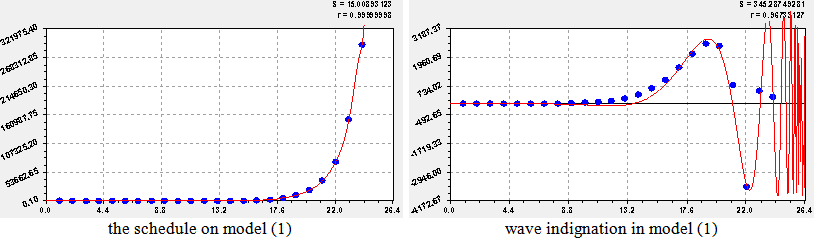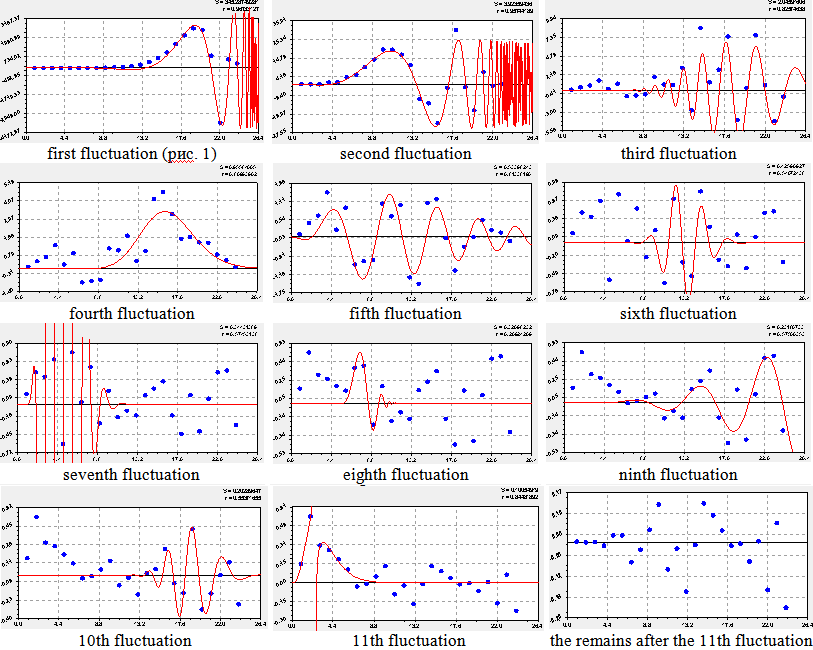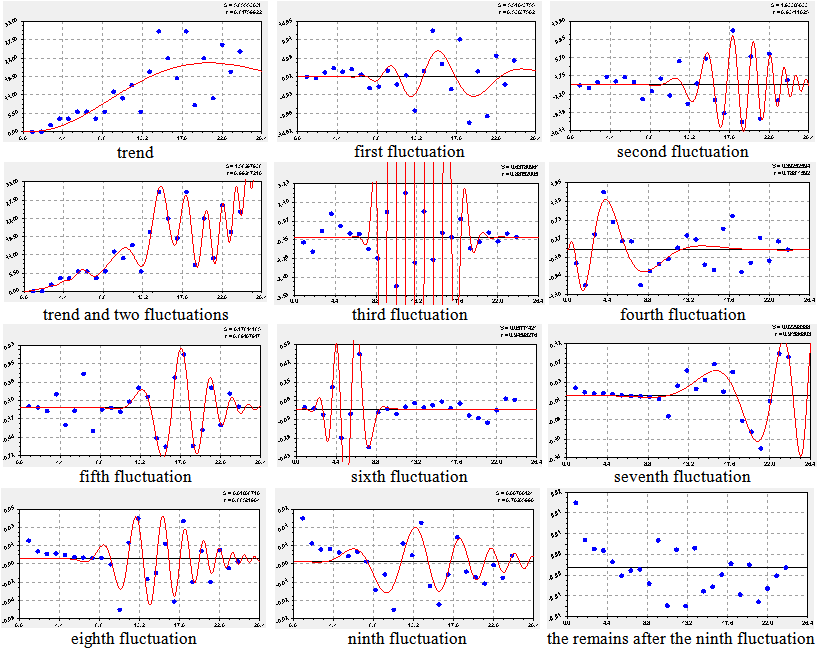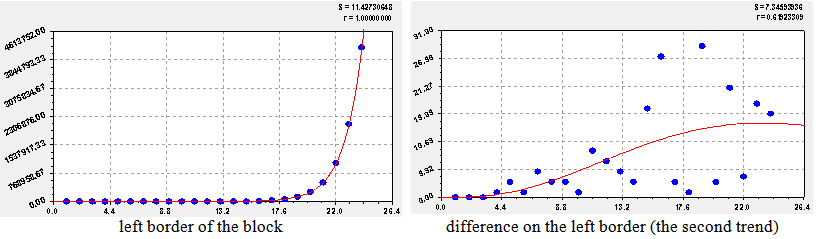## Figures index

#### P.M. Mazurkin

Applied Mathematics and Physics. 2014, 2(4), 146-156 doi:10.12691/amp-2-4-4• Figure 1. Schedules of power of blocks of binary decomposition of prime numbers• Figure 2. Schedules of model of dynamics of power of blocks of prime numbers• Figure 3. Schedules of members of model of dynamics of length of blocks of prime numbers• Figure 4. Schedules of members of model of dynamics of a growth of blocks of prime numbers• Figure 5. Schedules of the left border of blocks of binary decomposition of prime numbers• Figure 6. Schedules of wave members of model of dynamics of the left reference point of blocks of prime numbers• Figure 7. Schedules of wave members of model of dynamics of the right reference point of blocks of prime numbers• Figure 8. Center of symmetry of a number of WPN• Figure 9. Gain jump from 1 to 2 in a complete series of positive prime numbers